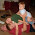Tuesday, February 8, 2011

Prime and composite numbers

The Transformation of a math teacher from one who “drilled and killed” to one who engages
Many teachers have commented that I talk a lot about theory but not enough about practicality.  I decided I will start focusing on how I changed my teaching style, through specific lessons, to allow for true engagement with my students.

Prime and composite numbers: The "old" way:
1) I would write the following definitions on the board:
Prime number – a number which has only two factors, 1 and itself
Composite number – a number which has more than 2 factors
2) I would then ask students to classify the following numbers as prime or composite 2, 4, 13….

3) I would then assign questions out of the textbook.
This is how I used to teach the difference between prime and composite.  Students found zero meaning and therefore never reached true engagement during this exercise.  Also, I had to assume that the students understood what a factor was, which usually took more time to redefine that term.   In all my years of teaching and studying mathematics I have never actually used the definitions of prime and composite, but instead required to apply what prime and composite numbers are.  If I, the master of the classroom, have never used the definition, why am I requiring my students to learn it?
This year I scrapped the definition of these two words and taught application and use; here is how:
I gave my students the following problem:
You are planning a wedding and you and your spouse are very particular about seating arrangements.  You can arrange the tables in any way as long as there is the same amount of people at each table.  You have a total of 100 people coming to your wedding, how can you arrange the tables?
(Usually I would start with a small number and increase the difficulty, but this year I have all my students sitting in groups where collaboration is promoted and therefore started with a larger number.)
My students started talking, “25 tables of 4 each, or 4 tables of 25 each, …..”, all groups even generated “1 group of 100 and 100 groups of 1”.  We then discussed reasonable sitting and why 1 group of 100 is unpractical (This discussion needs to occur to fully understand the application of prime and composite).  I then added the following sentence:
Your spouse forgot to invite her favourite uncle, now bringing the total 101.  Describe any problems and solutions.
At first students were confused.  They couldn’t find a number for which 101 could be broken up into.  After some thought, groups were starting to write “101 groups of 1 or 1 group of 101”.  Groups were commenting “I wouldn’t have 101 people; we would need to invite 3 more people so the total is 104 and have 13 tables of 8 each”.  I smiled!
Students understood the difference between these weird numbers that could not be broken up and the other ones where actual application could be used.  I concluded the lesson with the formal terms of prime and composite.
Application should be the focus of math class not formal definitions.  Math has beauty all throughout it, and the more we show off this beauty the more students will become engaged to learn math.

1.This is awesome, I love it. It reminds me of teaching remainders to my own kids. We go frisbee golfing (that's right, I said frisbee, not disc :) and each one wants to throw first. I told them to pick a remainder number (0, 1, or 2). We then divide the hole number by 3 and check the remainder. That kid gets to throw first.

2.Thanks for the great idea!

3.I am going to use this. Thanks for sharing this. If this isn't seen as practical, I don't know what could be.

You do good work.

4.This is awesome!!! Great way to turn a pretty "boring" concept into problem solving activity. This is what the new math curriculum is asking all math teachers to do. Thanks for sharing it. I am definitely going to use it.

5.Good to hear Sue, Joe, and Terry, let me know how it goes!

6.Great Idea. I think I'll try this with my 5th grade class. Thanks.

7.TeachMeWell - Online Math Help - education for children Aged 5 to 10 (Grade 1, Grade 2, Grade 3, Grade 4, Grade 5) - Math lessons click here math help

8.9.This idea is great! I will try it with my 4th grade class!

10.I just taught area of rectangles to my 5th graders. Then I gave them a worksheet and unifex cubes. The worksheet had columns labeled "Area of the rectangle" and "Dimensions of the rectangle." , They had to find all the dimensions they could for rectangles with areas of 1 square to 30 squares. If they were missing dimensions, I told them to find more.
Then highlight in yellow all the rectangles that could be arranged only one unique way (therefore, eliminating 1 x 2 and 2 x 1 as two possibles dimensions, since they were just transformations of the same rectangle).
Ask questions about why these rectangles only have one way of making dimensions with whole numbers. Then we went further and put green dots on all the rectangle areas that could be arranged as squares, and a red dot on the number 1.
We talked about factors, and how to list factors. Put a check next to all the rectangles that have an odd Number of factors. (They noticed that this related to all the numbers that had green dots, since they were square numbers).
After all this, I introduced the concept of prime and composite, and showed them the TED-ed Video called the "Can you solve the Locker Riddle?"